# NCERT Solutions - Gravitation, Science, Class 9 Notes | Study Class 9 Science by VP Classes - Class 9

## Class 9: NCERT Solutions - Gravitation, Science, Class 9 Notes | Study Class 9 Science by VP Classes - Class 9

The document NCERT Solutions - Gravitation, Science, Class 9 Notes | Study Class 9 Science by VP Classes - Class 9 is a part of the Class 9 Course Class 9 Science by VP Classes.
All you need of Class 9 at this link: Class 9

Q1. State the universal law of gravitation.
Ans.
Every object in the universe attracts every other object with a force which is proportional to the product of their masses and inversely proportional to the square of the distance between them. The force is along the line joining the centres of two objects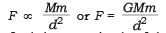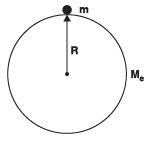Q2. Write the formula to find the magnitude of the gravitational force between the earth and an object on the surface of the earth.
Ans.
The gravitational force between the earth and an object on its surface

Me = Mass of the earth

R = Radius of the earth

m= Mass of an object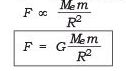∵ G = Gravitational constant

Q3. What do you mean by free fall?
Ans.
Whenever an object falls toward earth under the force of gravity alone and no other force is present, the motion of object is said to be “free fall”.

Q4. What do you mean by acceleration due to gravity?
Ans.
The acceleration of free fall is the acceleration due to gravity. We can also say the acceleration of an object due to gravitational force of earth acting on it is known as acceleration due to gravity.

Q5. What are the differences between the mass of an object and its weight? Ans.

 Mass Weight 1.Mass of a body is the measure of its inertia. 1.Weight of the body is the force with which it is attracted towards the earth (W = m × g). 2. Its S.I. unit is kg. 2. Its S.I unit is Newton. 3. It remains constant everywhere and it cannot be zero. 3. Its value changes from place to place and it can be zero. 4. It can be measured by beam-balance. 4. It can be measured by spring balance. 5. It has only magnitude i.e. it is a scalar quantity. 5. It has both magnitude and direction i.e. it is a vector quantity.

Q6. Why is the weight of an object on the moon 1/6 th its weight on the earth?
Ans.
The weight of an object depends on ‘g’ acceleration due to gravity, and the value of ‘g’ on earth and moon is not same.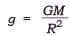The mass and radius of the earth is more than the mass and radius of the moon.

As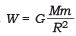the weight of a body on the earth is 6 times more than theweight of a same body on moon.

Q7. Why is it difficult to hold a school bag having a strap made of a thin and strong string?
Ans.
The force exerted by a thin and strong string is distributed to very less area and hence the force applied due to the bag is more, the pressure exerted on the body by thin straps will be more and hence will be more painful.
As pressure is inversely proportional to area, if the area is reduced pressure increases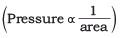Q8. What do you mean by buoyancy?
Ans.
The upward force exerted by any fluid (liquid, gas) on an object is known as upthrust or buoyancy.

Q9. Why does an object float or sink when placed on the surface of water?
Ans.
The density of the object and water decides the floating or sinking of the object in water.
The density of water is 1 gm/cm3.

• If the density of an object is less than the density of water then the object will float.
• If the density of an object is more than the density of water then the object will sink.

Q10. You find your mass to be 42 kg on a weighing machine. Is your mass more or less than 42 kg?
Ans.
The weighing machine actually measures the mass of the body. Hence the mass reading of 42 kg given by a weighing machine is same as the actual mass of the body. As mass is the quantity of inertia, it remains the same.

Q11. You have a bag of cotton and an iron bar, each indicating a mass of 100 kg when measured on a weighing machine. In reality, one is heavier than other. Can you say which one is heavier and why?
Ans.
The heaviness of the bag can be given by density

Density = Mass / volume

Mass of both cotton bag and iron bag is same. But the volume of cotton bag is more than the iron bag.
Hence density is inversely proportional to volume. The bag of iron will be heavier.

NCERT Textual Questions Solved

Q1. How does the force of gravitation between two objects change when the distance between them is reduced to half?
Ans.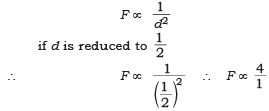The force of gravitation becomes 4 times more.

Q2. Gravitational force acts on all objects in proportion to their masses. Why then, a heavy object does not fall faster than a light object?
Ans.
The heavy object when falls, the acceleration due to gravity ‘g’ is acting on it, which is independent of the mass of the body.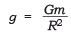Therefore, a heavy object doesn’t fall faster than a light object.

Q3. What is the magnitude of the gravitational force between the earth and a 1 kg object on its surface? [Mass of the earth is 6 × 1024 kg and radius of the earth is 6.4 × 106 m].
Ans.
The magnitude of the gravitational force between earth and an object is given by the formula.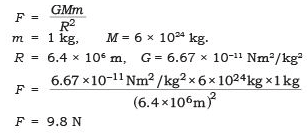Q4. The earth and the moon are attracted to each other by gravitational force. Does the earth attract the moon with a force that is greater or smaller or the same as the force with which the moon attracts the earth? Why?
Ans.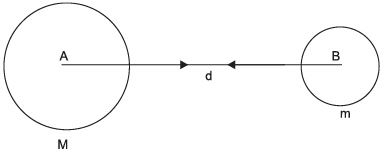Gravitational force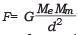The value of F is same for earth and the moon. Both the bodies will exert the same amount of force on each other.
As per universal law of gravitation, every body attracts the other body with some force and this force is same for both the bodies called gravitational force.

Q5. If the moon attracts the earth, why does the earth not move towards the moon?
Ans.
According to the universal law of gravitation both moon and earth attract each other with a force that is directly proportional to the product of their masses and inversely proportional to the square of distance between them. The force of attraction of moon on the earth is present, but the earth does not appear to move towards the moon as the mass of the earth is large and the distance between the moon and earth is so large, even if the earth is attracted/moves towards the moon it is negligible, cannot be seen.

Q6. What happens to the force between two objects, if
(i) the mass of one object is doubled?
(ii) the distance between the objects is doubled and tripled?
(iii) the masses of both objects are doubled?
Ans
. (i) If the mass of one object is doubled, the force between two objects will be doubled (increases)
(ii) If the distance between the objects is doubled the force between two objects will be one-fourth and if the distance will be tripled, the force will be one-ninth (1/9).
(iii) If the masses of both objects are doubled the force will be 4 times.
As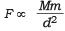Q7. What is the importance of universal law of gravitation?
Ans.
The universal law of gravitation explains several phenomena:
(i) it explains about the force that binds the earth,
(ii) the motion of the moon around the earth,
(iii) the motion of planets around the Sun, and
(iv) the tides due to the moon and the Sun.

Q8. What is the acceleration of free fall?
Ans.
The acceleration of free fall is, when the body falls due to earth’s gravitational pull, its velocity changes and is said to be accelerated due to the earth’s gravity and it falls freely called as free fall. This acceleration is calculated to be 9.8 m/s2.

Q9. What do we call the gravitational force between the earth and an object?
Ans.
The gravitational force between the earth and an object is called force due to gravity. It is measured as the weight of an object.

Q10. Amit buys few grams of gold at the poles as per the instruction of one of his friends. He hands over the same when he meets him at the equator. Will the friend agree with the weight of gold bought? If not, why? [Hints: The value of g is greater at the poles than at the equator.]
Ans.
Weight of the body is given by the formula W = mg It depends on the value of ‘g’ i.e., acceleration due to gravity.
The weight of gold at poles = Wp = m × g (poles) Value of g at poles is more than the value of g at equator.
The weight of gold at equator = We = m × g (equator)
∴           Wp > We.
The weight at pole of the same gold is found to be more as compared to the weight at the equator. Hence, the friend will not agree with the weight of the gold bought.

Q11. Why will a sheet of paper fall slower than one that is crumpled into a ball?
Ans.
A sheet of paper has larger surface area and while falling down it has to overcome the force exerted by air/wind current called as air resistance.
The crumpled paper has smaller surface area and it has to overcome very less amount of air current.
The air resistance offered to plain sheet is more than the resistance to crumpled sheet. Hence, the sheet of paper fall slower than the crumpled one.

Q12. Gravitational force on the surface of the moon is only 1/6 as strong as gravitational force on the earth. What is the weight in Newtons of a 10 kg object on the moon and on the earth?
Ans.
Mass of the object = 10 kg Weight of the object on earth = W = m × g
∴ W = 10 × 9.8
W = 98 N
Weight of the object on moon = (1/6)th the weight on the earth.
As the gravitational force on the surface of the moon is only (1/6) th as strong as gravitational force on the surface of the earth.
∴ Weight of the object on moon = (98/6)= 16.3 N
Weight on earth = 98 N
Weight on moon = 16.3 N

Q13. A ball is thrown vertically upwards with a velocity of 49 m/s. Calculate
(i) the maximum height to which it rises,
(ii) the total time it takes to return to the surface of the earth.
Ans.
(i) Initial velocity,
u = 49 m/s Final velocity,
v = 0 m/s a = g = − 9.8 m/s(going against the gravity)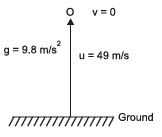Height (h) = Distance = s = ?
∴   v2 − u2 = 2gs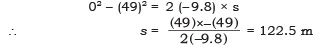(ii) Time taken, t = ? v = u + gt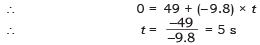Total time taken to return the surface of the earth by the ball is 5 s + 5 s = 10 s.

Q14. A stone is released from the top of a tower of height 19.6 m. Calculate its final velocity just before touching the ground?
Ans.
Data u = 0 m/s
v = ?
h = s = 19.6 m
g = 9.8 m/s2 (falling down)
v2 − u2 = 2gs
v− (0)2 = 2 × 9.8 × 19.6
∴ v2 = 19.6 × 19.6
∴ v = 19.6 m/s
The final velocity just before touching the ground is 19.6 m/s.

Q15. A stone is thrown vertically upward with an initial velocity of 40 m/s. Taking g = 10 m/s2, find the maximum height reached by the stone. What is the net displacement and the total distance covered by the stone?
Ans.
u = 40 m/s
g = − 10 m/s2 (going against gravity)
h = s = ?
v = 0
v2 − u2 = 2gs
(0)2 − (40)2 = 2 (− 10) × s

∴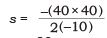∴     s = 80 m

Net displacement of the stone = 0 (As the stone falls, back to the same point.) Total distance covered by stone = 80m up  +  80m down
= 160 m

Q16. Calculate the force of gravitation between the earth and the Sun, given that the mass of the earth = 6 × 1024 kg and of the Sun = 2 × 1030 kg. The average distance between the two is 1.5 × 1011 m.
Ans.
Me = 6 × 1024 kg,
G = 6.67 × 10−11 Nm2/kg2
Ms = 2 × 1030 kg
d = 1.5 × 1011 m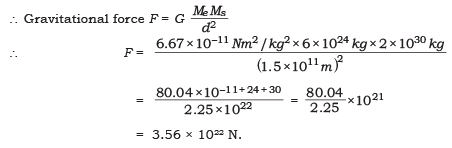Q17. A stone is allowed to fall from the top of a tower 100 m high and at the same time another stone is projected vertically upwards from the ground with a velocity of 25 m/s. Calculate when and where the two stones will meet.
Ans.
h = 100 m time,
t = ?
g = 10 m/s2
Height covered by the falling stone = s1
∴ s1 = ut + (1/2)gt2
∴   s1 = 0 × t + (1/2) (10) t 2

∴   s1 = 5t              ...(1)
The distance covered by the stone thrown upward = s2
g = − 10 m/s
u = 25 m/s
s2 = ut + (1/2) gt2
∴  s2 = 25t + (1/2) (− 10)t 2
∴ s2 = 25t − 5t2 ...(2)
Total height given = 100 m
∴   s1 + s2 = 100 m 5t+ (25t − 5t2) = 100 m
∴   25t = 100 m
t = 25
100 = 4 seconds ...(3)
Putting the value of equation (3) in equation (1), we get ∴
s1 = 5t2 = 5 × (4)2 = 80 m
∴ The two stones will meet after 4 seconds when the falling stone has covered a height of 80 m.

Q18. A ball thrown up vertically returns to the thrower after 6 s. Find
(a) the velocity with which it was thrown up,
(b) the maximum height it reaches, and
(c) its position after 4 s.
Ans.
u = ?
v = 0
g = − 9.8 m/s(thrown upward)
Total time = 6s (to go up and down)
∴ Time for upward journey =(6/2) = 3 s.

(a) v = u + gt
0 = u + (− 9.8) × 3
u = 29.4 m/s

(b) Maximum height, h = s = ?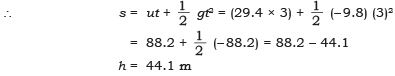(c) Position after 4 s t = 4 s
∴ s = ut + (1/2) gt2
s = (29.4 × 4) +(1/2) (− 9.8) × (4)= 117.6 + (1/2)(− 156.8) = 117.6 − 78.4 = 39.2 m.
∴ Position after 4 s = 39.2 m from the top.

Q19. In what direction does the buoyant force on an object immersed in a liquid act?
Ans.
The buoyant force on an object immersed in a liquid acts upwards, i.e. opposite to the direction of the force exerted by the object.

Q20. Why does a block of plastic released under water come up to the surface of water?
Ans.
The floating or sinking of a body in the water is decided by the density of both the body and water’s buoyant force acting on the body.
The density of plastic is less than the water and the buoyant force exerted by water on the plastic block is greater than the force exerted by plastic on the water.

Q21. The volume of 50 g of a substance is 20 cm3. If the density of water is 1 g cm–3, will the substance float or sink?
Ans.
Mass = 50g
Volume = 20 cm3

Density = mass/ volume

∴ Density of a given substance =   50g/ 20 cm3 = 2.5g/cm3

Density of water = 1 g/cm3 As the density of a given substance is more than the density of water. The substance will sink in water.

Q22. The volume of a 500 g sealed packet is 350 cm3. Will the packet float or sink in water if the density of water is 1g cm–3? What will be the mass of the water displaced by this packet?
Ans.
Mass of the packet = 500 g
Volume of the packet = 350 cm3
Density of the packet = ?
Density =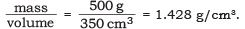Density of water = 1 g/cm3
The packet will sink in water as the density of packet is greater than the density of water.
Mass of the water displaced by this packet = Volume of the packet × Density of water = 350 cm3 × 1 g/cm3 = 350 g

The document NCERT Solutions - Gravitation, Science, Class 9 Notes | Study Class 9 Science by VP Classes - Class 9 is a part of the Class 9 Course Class 9 Science by VP Classes.
All you need of Class 9 at this link: Class 9Use Code STAYHOME200 and get INR 200 additional OFF

## Class 9 Science by VP Classes

75 docs|14 tests

Track your progress, build streaks, highlight & save important lessons and more!

,

,

,

,

,

,

,

,

,

,

,

,

,

,

,

,

,

,

,

,

,

,

,

,

,

,

,

;